# How to find a formula for the month in excel (easy steps)

July 22, 2022
532 Views
0

A free Office suite fully compatible with Microsoft OfficeThe spreadsheet program is valuable these days and is generally used by entire company workers. It makes work easier for employees and with the help of these functions and programs they work smartly and even in this busy world it saves a lot of time for a human being. This function is useful in many manners like to get product details of expiry, employees salary month and much more.

To find a formula for the month in excel we use the following formula by which we easily get the result successfully.

Formula: =MONTH(date)

There are several steps by which we find the formula of the month easily.

1) The first step is to make data on which we will use the following formula.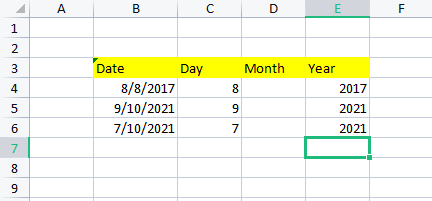2) In The second step, here we will add the formula in D section D4 and after implying the formula press enter key so automatically a number of the month will appear in the box.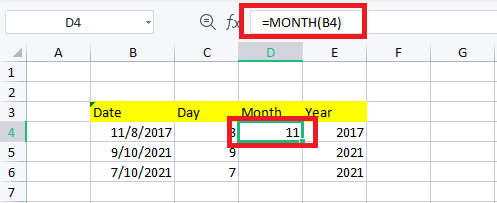As you find in the above-attached picture number 11 is highlighted because of the formula we use in D4.

3) Our last step is to copy the formula up to D6 and automatically all the values of the months will appear in their boxes.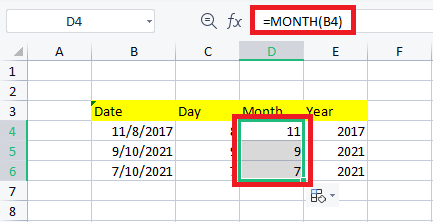Congratulations you successfully find the formula of the month in excel and after implying it in the month column you get your results also.

By following all these steps respectfully, you will find the formula for the month in the spreadsheet easily.

How to find months by using the EDATE function in Excel.

In excel we can easily add months using the EDATE function. This can be very useful for any company in their financial phases and modeling where they can easily adjust their time in actual periods. There are some steps by which we can add a month in excel.

=EDATE(start date, number of months). This formula we use to add months to a spreadsheet.

1) First we have to start with the essentials and their elements.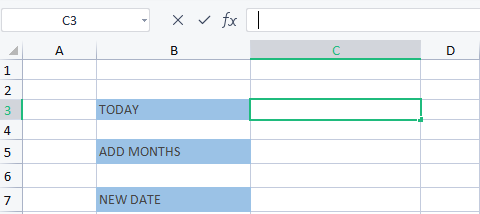2) Now we have to place the formula in the C3 of =TODAY(), by this you get today’s date.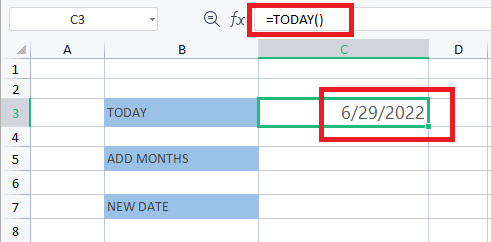3) After getting the current date we have to add months in C5 and after that imply the formula to get the result.

1:In this below example we added months in it to determine the result.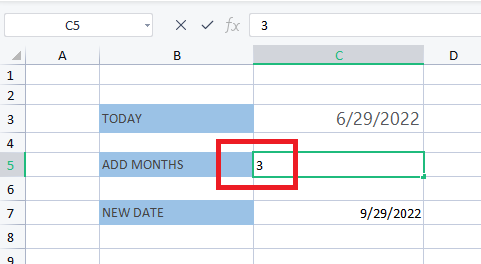2: Now we place the EDATE formula in C7 to get the added month.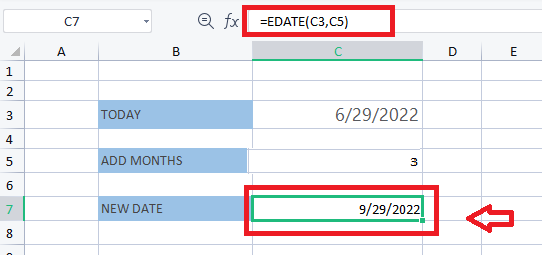It is as easy as that, So, finally, with all these steps and processes we find how to add a month in excel.

How to Add Months in Excel

Using MONTH & Date Function

This Excel function is used when we need to take three separate values to merge them to form a date, this function is also used in companies to look after their monthly period.

There are a few steps to get to know how to add months to date in spreadsheets. Now for showing this method we have to consider some examples.

1) The First step is to follow some elements in it.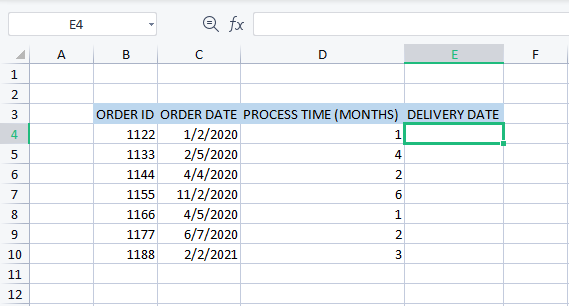2) After this we have to place the formula of EDATE which can be useful to get the results.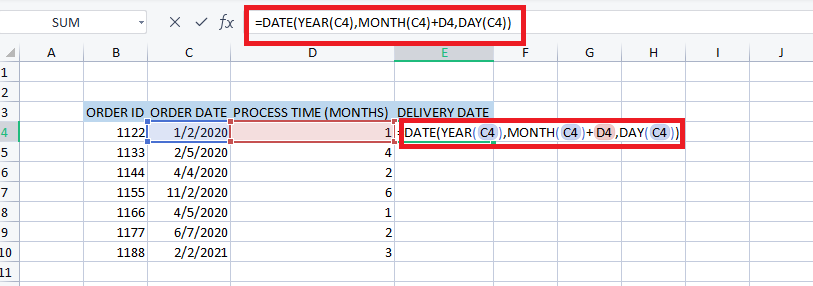3) After pressing Enter key the date will appear in E4 and after that, copy the formula up to the last E10 so all the dates will appear in their boxes.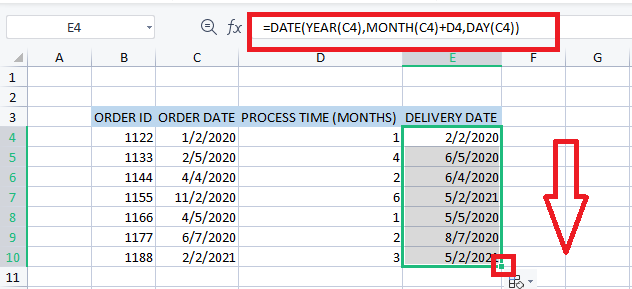By following all these steps we find how to add months to date in Excel using the month and date function. This is the easiest and most interesting way to evaluate it.

You can also find this feature in all the latest excel versions 2016/2018/2019 spreadsheet, where you can easily find the formula for a month in excel.